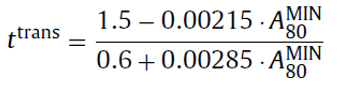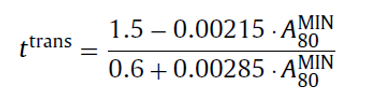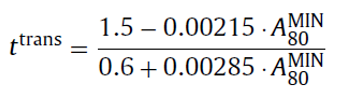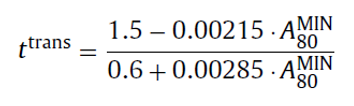# Forming Limit Curve (FLC)

The stress-strain curve of a material illustrates its mechanical properties and gives the forming limit beyond which it fails. Inspire Form supports several methods for calculating the stress-strain curve.

## Keeler – Beizer (Original)

Inputs : Sheet thickness (t), Strain hardening (n).

## Keeler – Beizer (Modified I)

Inputs : Sheet thickness (t), Strain hardening (n).

## Keeler – Beizer (Modified II)

Inputs : Sheet thickness (t), Strain hardening (n).

Inputs : Sheet thickness (t), Strain hardening (n).

## Abspoel & Scholting

Inputs : Sheet thickness (t), Strain hardening (n), Total elongation in 0 degree (A80), Min Total elongation among all directions and Average Anisotropy (r).

Method : Calculate four points to create the FLC.
Point 1: TE
No thickness dependency for the validity of the equation.
Point 2: PS
No thickness dependency for the validity of the equation.
Point 3: IM
• If thickness (t) is less than or equal to• If thickness is greather thanPoint 4: BI
• If thickness (t) is less than or equal to• If thickness is greather than